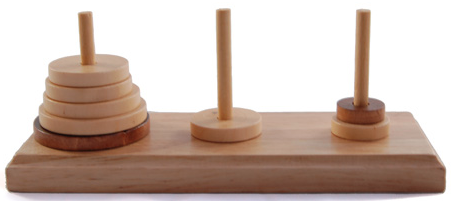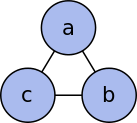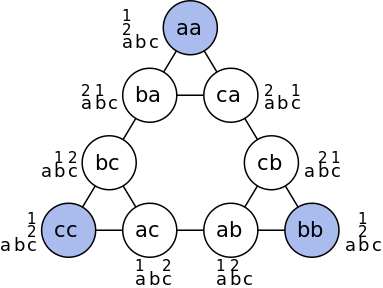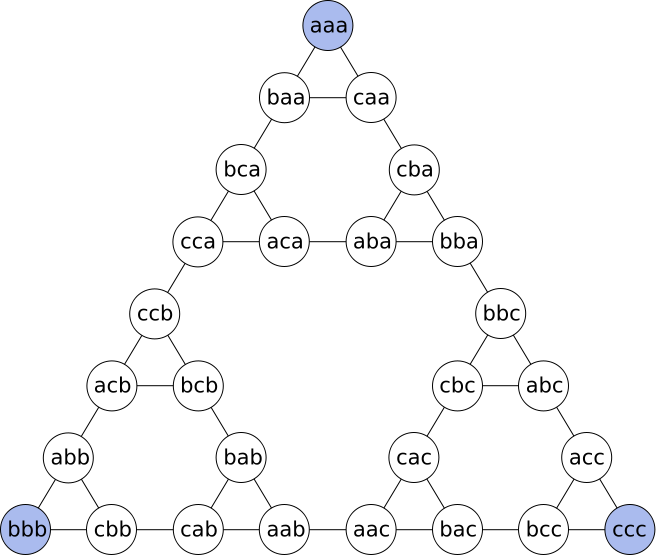# 汉诺塔问题# 解决和证明

• 初始柱子：最开始时，所有圆盘所处的柱子
• 目标柱子：最终完成时，所有圆盘所处的柱子
• 中间柱子：除了初始柱子和目标柱子以外的柱子

## 给出一种递归解

$$T_n\leq2T_{n-1}+1$$

## 证明递归解最优

$$T_n\geq2T_{n-1}+1$$

$$T_n=\begin{cases}0&n=0\\ 2T_{n-1}+1&n>0\\ \end{cases}$$

# 变种问题

• 有 $2n$ 个盘子，共有 $n$ 种大小的尺寸，每种尺寸都为两个，而且同样大小的盘子不区分。这种情况最少需要移动多少次？
• 条件同上，但是同样大小的盘子需要区分，移动到目标柱子上后需要保持和原先一样的上下顺序。这种情况最少需要移动多少次？
• 初始柱子和目标柱子之间不再能够直接移动，必须先移动到中间柱子中转。这种情况下最少需要移动多少次？

# 任意状态转移# 四个柱子的情况

## 给出一种递归解

$$M_n\leq2M_k+T_{n-k}$$

$$k=n-round\left(\sqrt{2n+1}\right)+1$$

# 通用的问题描述

## Frame-Stewart算法

$$T\left(n,r\right)=2T\left(k,r\right)+T\left(n-k,r-1\right)$$

$k$ 的取值公式同上。对于 $r>4$ 的情况都是还未得到证明的。